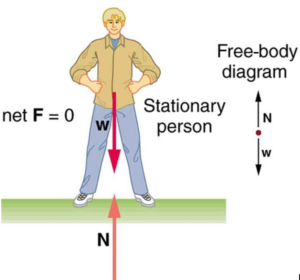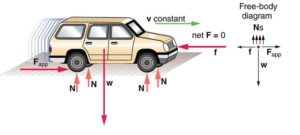Blog / Vector Analysis Of Forces Acting On A Point Object

### Vector analysis of forces acting on a point object

Written by Chau Pham on Feb 26, 2020

For an object to be in equilibrium, it must be experiencing no acceleration. This means that both the net force and the net torque on the object must be zero.

Here we will discuss the first condition, that of zero net force. If net force is zero, then net force along any direction is zero. The forces acting along each axis of motion must sum to zero: the net external forces along the typical x– and y-axes are zero.

This is true for both static equilibrium, where the object’s velocity is zero, and dynamic equilibrium, where the object is moving at a constant velocity.

Below, the motionless person is in static equilibrium. The forces acting on him add up to zero. Both forces are vertical in this case.In another case, the car is in dynamic equilibrium because it is moving at constant velocity. There are horizontal and vertical forces, but the net external force in any direction is zero. The applied force between the tires and the road is balanced by air friction, and the weight of the car is supported by the normal forces, here shown to be equal for all four tires.### KEY TAKEAWAYS

#### Key Points

• There are two conditions for an object to be in equilibrium.
• The first condition is that the net force on the object must be zero for the object to be in equilibrium.
• An object can be in static or dynamic equilibrium.

#### Key Terms

• Force: A physical quantity that denotes ability to push, pull, twist or accelerate a body which is measured in a unit dimensioned in mass × distance/time² (ML/T²): SI: newton (N); CGS: dyne (dyn)
• Zero net force: Forces equal in magnitude but acting in opposite directions that cancel one another out

Billing Information